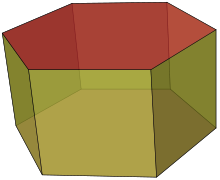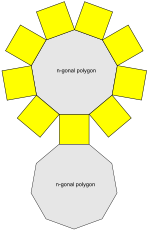Prism (geometry)

﻿
Prism (geometry)
Set of uniform prisms(A hexagonal prism is shown)
Type uniform polyhedron
Faces 2+n total:
2 {n}
n {4}
Edges 3n
Vertices 2n
Schläfli symbol {p}x{} or t{2, n}
Coxeter-Dynkin diagramVertex configuration 4.4.n
Symmetry group Dnh, [n,2], (*22n), order 2n
Dual polyhedron bipyramids
Properties convex, semi-regular vertex-transitiven-gonal prism net (n = 9 here)

In geometry, a prism is a polyhedron with an n-sided polygonal base, a translated copy (not in the same plane as the first), and n other faces (necessarily all parallelograms) joining corresponding sides of the two bases. All cross-sections parallel to the base faces are the same. Prisms are named for their base, so a prism with a pentagonal base is called a pentagonal prism. The prisms are a subclass of the prismatoids.

General, right and uniform prisms

A right prism is a prism in which the joining edges and faces are perpendicular to the base faces. This applies if the joining faces are rectangular. If the joining edges and faces are not perpendicular to the base faces, it is called an oblique prism.

Some texts may apply the term rectangular prism or square prism to both a right rectangular-sided prism and a right square-sided prism. The term uniform prism can be used for a right prism with square sides, since such prisms are in the set of uniform polyhedra.

An n-prism, having regular polygon ends and rectangular sides, approaches a cylindrical solid as n approaches infinity.

Right prisms with regular bases and equal edge lengths form one of the two infinite series of semiregular polyhedra, the other series being the antiprisms.

The dual of a right prism is a bipyramid.

A parallelepiped is a prism of which the base is a parallelogram, or equivalently a polyhedron with 6 faces which are all parallelograms.

A right rectangular prism is also called a cuboid, or informally a rectangular box. A right square prism is simply a square box, and may also be called a square cuboid.

An equilateral square prism is not a cube.

Volume

The volume of a prism is the product of the area of the base and the distance between the two base faces, or the height (in the case of a non-right prism, note that this means the perpendicular distance).

The volume is therefore:$V = B \cdot h$

where B is the base area and h is the height. The volume of a prism whose base is a regular n-sided polygon with side length s is therefore:$V = \frac{n}{4}hs^2 \cot\frac{\pi}{n}.$

Surface area

The surface area of a right prism is$2\cdot B + P\cdot h$, where B is the area of the base, h the height, and P the base perimeter.

The surface area of a right prism whose base is a regular n-sided polygon with side length S and height h is therefore:$A = \frac{n}{2} S^2 \cot{\frac{\pi}{n}} + n S h.$

Symmetry

The symmetry group of a right n-sided prism with regular base is Dnh of order 4n, except in the case of a cube, which has the larger symmetry group Oh of order 47, which has three versions of D4h as subgroups. The rotation group is Dn of order 2n, except in the case of a cube, which has the larger symmetry group O of order 24, which has three versions of D4 as subgroups.

The symmetry group Dnh contains inversion iff n is even.

Prismatic polytope

A prismatic polytope is a dimensional generalization of a prism. An n-dimensional prismatic polytope is constructed from two (n − 1)-dimensional polytopes, translated into the next dimension.

The prismatic n-polytope elements are doubled from the (n − 1)-polytope elements and then creating new elements from the next lower element.

Take an n-polytope with fi i-face elements (i = 0, ,..., n). Its (n + 1)-polytope prism will have 2fi + fi−1 i-face elements. (With f−1 = 0, fn = 1.)

By dimension:

• Take a polygon with n vertices, n edges. Its prism has 2n vertices, 3n edges, and 2 + n faces.
• Take a polyhedron with v vertices, e edges, and f faces. Its prism has 2v vertices, 2e + v edges, 2f + e faces, and 2 + f cells.
• Take a polychoron with v vertices, e edges, f faces and c cells. Its prism has 2v vertices, 2e + v edges, 2f + e faces, and 2c + f cells, and 2 + c hypercells.

Uniform prismatic polytope

A regular n-polytope represented by Schläfli symbol {pq, ..., t} can form a uniform prismatic (n + 1)-polytope represented by a Cartesian product of two Schläfli symbols: {pq, ..., t}x{}.

By dimension:

Higher order prismatic polytopes also exist as Cartesian products of any two polytopes. The dimension of a polytope is the product of the dimensions of the elements. The first example of these exist in 4-dimensional space are called duoprisms as the product of two polygons. Regular duoprisms are represented as {p}×{q}.

Wikimedia Foundation. 2010.

Look at other dictionaries:

• Prism — may refer to:cience and mathematics* Prism (optics), a transparent object which refracts light ** Dispersive prism, the most familiar type of optical prism * Prism (geometry), a kind of polyhedron * Prism (geology), a kind of sedimentary… …   Wikipedia

• Prism (optics) — For other uses, see Prism (disambiguation). A plastic prism In optics, a prism is a transparent optical element with flat, polished surfaces that refract light. The exact angles between the surfaces depend on the application. The traditional… …   Wikipedia

• prism — ► NOUN 1) a piece of glass or other transparent material of regular shape, used to separate white light into a spectrum of colours. 2) Geometry a solid geometric figure whose two ends are similar, equal, and parallel rectilinear figures, and… …   English terms dictionary

• Prism compressor — A prism compressor is an optical device used to shorten the duration of a positively chirped ultrashort laser pulse by giving different wavelength components a different time delay. It typically consists of two prisms and a mirror. Figure 1 shows …   Wikipedia

• prism — /ˈprɪzəm / (say prizuhm) noun 1. Optics a transparent body (especially one with triangular bases) used for decomposing light into its spectrum or for reflecting light beams. 2. Geometry a solid whose bases or ends are any congruent and parallel… …   Australian English dictionary

• prism — prÉªzm n. piece of transparent glass or crystal with triangular bases (used to separate light into the colors of the spectrum); solid figure whose ends are equal and parallel polygons and whose faces are parallelograms (Geometry) …   English contemporary dictionary

• prism — [ prɪz(ə)m] noun 1》 Geometry a solid geometric figure whose two ends are similar, equal, and parallel rectilinear figures, and whose sides are parallelograms. 2》 Optics a transparent object in this form, especially one with triangular ends used… …   English new terms dictionary

• Triangular prism — In geometry, a triangular prism or three sided prism is a type of prism; it is a polyhedron made of a triangular base, a translated copy, and 3 faces joining corresponding sides.If the sides are squares, it is called a uniform polyhedron. In… …   Wikipedia

• Multiple-prism dispersion theory — The first description of multiple prism arrays, and multiple prism dispersion, was given by Newton in his book Opticks. Prism pair expanders were introduced by Brewster in 1813. A modern mathematical description of the single prism… …   Wikipedia

• Cylinder (geometry) — A right circular cylinder A cylinder is one of the most basic curvilinear geometric shapes, the surface formed by the points at a fixed distance from a given line segment, the axis of the cylinder. The solid enclosed by this surface and by two… …   Wikipedia